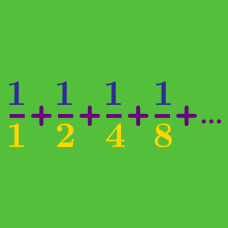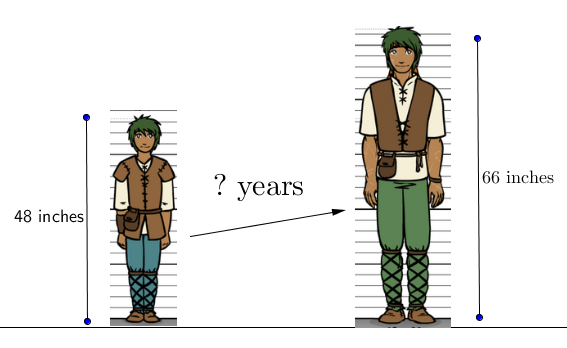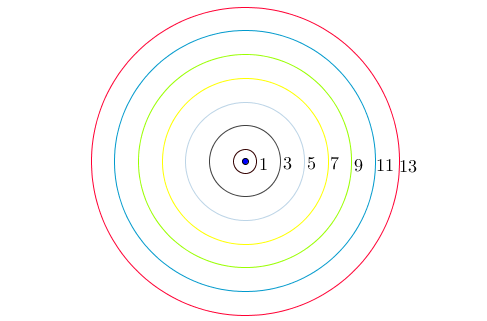Calculus

# Arithmetic Progressions

Which of the following sequences is an Arithmetic Progression?Suppose that when you are a teenager, your height increases at a steady rate of 3 inches every year. If your present height is 48 inches, then how many years will you take to grow to 66 inches tall?The figure shows the concentric circles with radii $1, 3,5,7, \ldots$ and so on. Find the area of 18th circle.

In the arithmetic progression $-110, -107, -104, \cdots,$ the $n^\text{th}$ term $a_n$ is the first positive number. What is $n$?

A sequence $\{a_n\}$ is defined as $a_1=12, a_{n+1}=a_n+3,$ where $n \in \mathbb{N}.$ What is the general term of $\{a_n\} ?$

×